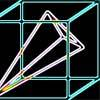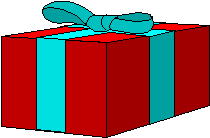#### You may also like### Plane to See

P is the midpoint of an edge of a cube and Q divides another edge in the ratio 1 to 4. Find the ratio of the volumes of the two pieces of the cube cut by a plane through PQ and a vertex.### Four Points on a Cube

What is the surface area of the tetrahedron with one vertex at O the vertex of a unit cube and the other vertices at the centres of the faces of the cube not containing O?### Instant Insanity

We have a set of four very innocent-looking cubes - each face coloured red, blue, green or white - and they have to be arranged in a row so that all of the four colours appear on each of the four long sides of the resulting cuboid.A box of size $a$ cm by $b$ cm by $c$ cm is to be wrapped with a square shaped piece of wrapping paper. Cutting the paper is not allowed so what is the smallest square this sheet can be?